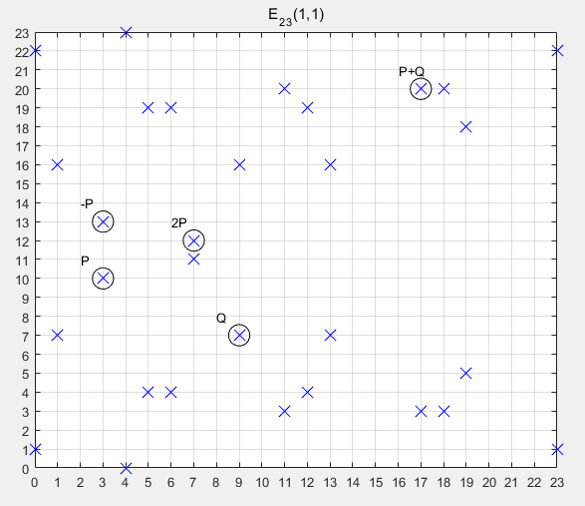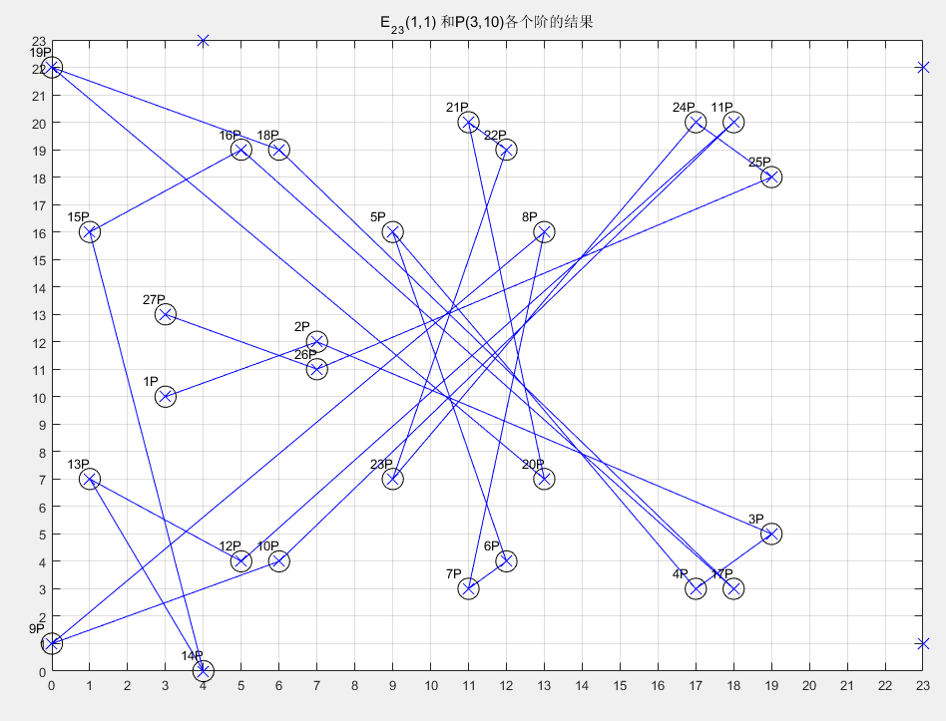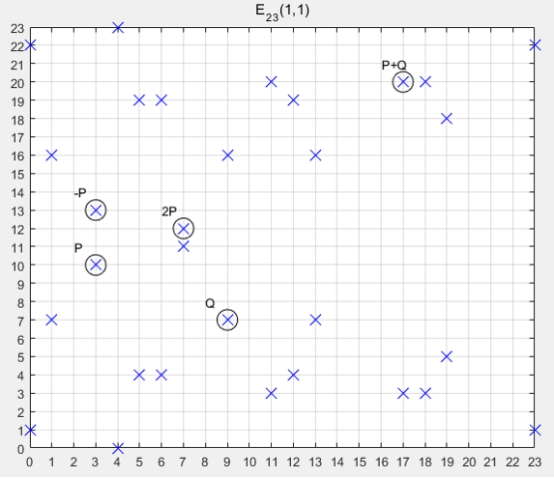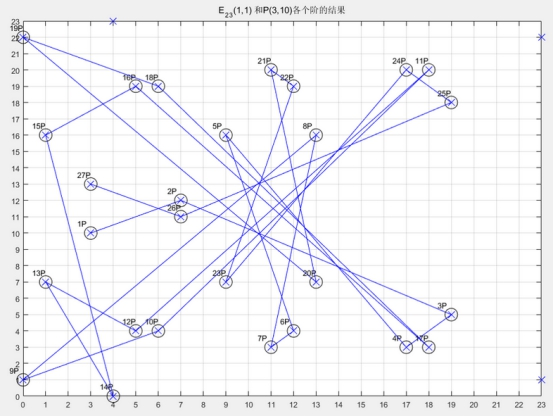• 的椭圆曲线化学组 我的Python代码，用于在有限域上试验椭圆曲线和椭圆曲线密码学。 该代码仅用于实验和教育目的，不用于现实世界的加密应用程序。 实验 短Weierstrass，Edwards，Twisted Edwards和Montgomery形状...Python
• 当然，后面提供的一些关于有限域上的椭圆曲线上的点的加法，乘法，除法的实装函数都是可以直接用于加解密的。这次要研究的ECPP，它是接我以前的一篇关于素数的blog...
要说明的是，现在这篇东西是研究用椭圆曲线精确判定一个正整数是否为素数，而不是研究用椭圆曲线加解密的！
当然，后面提供的一些关于有限域上的椭圆曲线上的点的加法，乘法，除法的实装函数都是可以直接用于加解密的。
这次要研究的ECPP，它是接我以前的一篇关于素数的blog（http://blog.csdn.net/chen09/archive/2008/03/24/2214219.aspx
），“试除法”以外的素数精确判定的方法之一，它的算法复杂度为：O((log n)^6)。

“有限域上的椭圆曲线”，这个概念的子概念，顾名思义有两个：1，有限域，2，椭圆曲线。这两个子概念都很容易理解，但是合在一起，定义在有限域上的椭圆曲线，而不是定义在普通的实数上的椭圆曲线，是怎么一回事儿？我大概搞了整整一个月才明白。网上的中文和日文资料都太少了，而且错误百出，让我走了不少弯路。在这里想把我所知道的写清楚，让后来人可以多一份可以参考的资料。

什么是椭圆曲线？什么是有限域？什么是定义在有限域上的椭圆曲线？它有什么特征？和判断素数有什么关系？
这里有一篇《ECC加密算法入门介绍》（http://www.docin.com/p-853208.html
），写得非常的好，错误几乎没有。通读一边有益无损。
初中时，我们就学过，方程x^2+y^2=1是个圆，以(0,0)为中心，半径为1。
稍作变形，方程(x-a)^2+(y-b)^2=c^2也是个圆，以(a,b)为中心，半径为c。
然后说说椭圆方程，(x/a)^2+(y/b)^2(a>b>0)是个标准方程。
稍作变形，((x-h)/a)^2+((y-k)/b)^2=1。h,k自然是x轴和y轴上的位移了。
有没有都回想起来呢？如果回想起来了，那么把它们都忘了吧！因为椭圆曲线和椭圆方程没有什么关系。8-)

《ECC加密算法入门介绍》（http://www.docin.com/p-853208.html
）里面讲椭圆曲线时先介绍了射影平面，这个有点超纲（在我看来，所有计算机的题目都是用初中数学可以解决的，凡是用到高中数学以上的东西，都是超纲的）。所以还是直接考察笛卡尔坐标系上的，椭圆曲线：y^2+a1*x*y+a3*y=x^3+a2*x^2+a4*x+a6。
看上去有点复杂？其实已经很简单化了！要知道：两个元（x和y）且是3次的一般方程要复杂的多。而现在要求每个点都是光滑的才是椭圆曲线，所以方程的形式真的已经简单很多。
还是觉得有点复杂？没关系，实际运用中的椭圆曲线的形式将更为简单。

现在，这个椭圆曲线是定义在实数上的，对于曲线上的点都可以求导（因为我们要求它是光滑的），求导的方法很简单，两边分别对y和x求偏导就可以。求导自然是为了就切线，为什么要算切线？稍微过一会儿说。

现在说一下，曲线上的2个点的加法。
什么是加法？
大家都知道1+1=2(不是哥德巴赫猜想，是小学或者说幼儿园的数学)，但是1+1为什么等于2？因为这个“+”是人为定义的一个算法，在首先自然数上定义，然后扩展到整数，有理数，实数，复数。

罗素（Bertrand Russell, 1872-1970）和他的导师怀特黑(Alfred Whitehead, 1861-1947)用了几十页的篇幅，最终成功地证明1+1=2（参见，若奇的《孤独的破译者和他的计算机器》 http://xys4.dxiong.com/xys/netters/psi1b/ruoqi.txt
）。

现在，天才的数学家们也定义了椭圆曲线上的两个点的加法！
令椭圆曲线两个点P，Q，做一条过点P和点Q的直线（如果P，Q重合，则做P点上的切线（这就是刚才说到为什么要求导求切线的原因）），交椭圆曲线上的另一点R'，过R'做y轴的平行线交椭圆曲线与点R。规定：P+Q=R!

为什么要定义这么个不着边际的运算法则？
的确，它离我们的目标，无论是精确素数判断还是费马大定理都离得很远。
这里，我想可以运用人择原理：如果它是没有用的，那么就不会被我们注目；虽然它不是那么直观，但是它是有用的，所以我们现在在学习它。

就好象，1+1=2，原来也许没有什么用，但是要计算1个苹果加上1个生梨，共有几个水果时，起到了作用，所以1+1=2被保留了下来。
1+1威力如此之大，不但用于幼儿的数学启蒙，也创造了诺贝尔奖获得者罗素！大家都知道诺贝尔是没有数学奖的，罗素得到的是文学奖。罗素和怀特黑的两千多页的《数学原理》（和牛顿的伟大的著作同名，说明他们有如此的自信）耗尽了他的心血，哥德尔(Kurt G?del, 1906-1978)和他的“哥德尔不完备定理”的出现，使罗素则彻底丧失了对数理逻辑的兴趣，他改而倾心哲学和文学，关注社会事务。终于，在1950年他获得了诺贝尔文学奖。

1+1威力还不止于此，同样因为哥德尔的出现，也使冯·诺依曼(John von Neumann, 1903-1957)放弃了纯粹数学的研究。1944年，他与奥斯卡·摩根斯特恩合著出版《博弈论与经济行为》，标志着现代系统博弈理论的的初步形成，从而被称为“博弈论之父”；1945年6月，他戈德斯坦、勃克斯等人联名发表了著名的“101页报告”，而成了现代计算机之父。

哥德尔是有颠覆性的，他的不完备定理最初可以描述为“如果将算术运算公理引入一阶谓词系统，则由此而成的算术运算系统是不完备的，即存在这样的命题，它们的真伪是不可证明的。”而一阶谓词显然与50年后（20世纪70，80年代）的计算机届有直接的关系，以日本为首的推崇的第5代计算机就是以Prolog为主要语言，而Prolog就是以一阶谓词逻辑为基础的。虽然第5代计算机以失败而告终，但是不得不佩服日本敢为天下先的勇气，如果成功，那么我们现在的世界也许完全不是现在这个样子的了。

扯了半天，想说明的就是，P+Q=R!

定义在实数上的椭圆加法，还有图像可以看，但是定义在有限域上的椭圆曲线及其加法，就很难理解了。杨振宁曾说过，现在的数学论文，有的是看了第一页就看不下去的；有的是看了第一句就看不下去的。幸亏，有限域上的椭圆曲线还是可以看下去的，虽然它比“1个苹果加上1个生梨”要复杂些。但是只要有了抽象的头脑，它并不比“2头牛加上3头羊等于5头动物”要难多少。

刚才，我们把椭圆曲线定义在实数上，它是连续的。现在我们把它定义在有限域上。
有限域的定义很简单：包含有限个元素的域被称为有限域。

如果从有限域扯开去，那么和我们熟知的一些数学和计算机都有很大关系。比方说，每个方程对应于一个域，即含有方程全部根的域，称为这方程的伽罗瓦域，这个域对应一个群，即这个方程根的置换群，称为这方程的伽罗瓦群。伽罗瓦域的子域和伽罗瓦群的子群有一一对应关系；当且仅当一个方程的伽罗瓦群是可解群时，这方程是根式可解的。
这个理论是不是很难理解？没有关系，它的推论就容易理解的多：1，可以得出五次以上一般代数方程沒有公式解（华罗庚曾经指出过某人的5次方程公式解的错误）；2，尺规作图三等分任意角和作倍立方体不可能；等等。

不过我们不需要知道那么多。我们只要知道定义在有限域上的椭圆曲线就是：
那些点P或者Q的坐标(x,y)都不是实数范围那么大，而是有限的。比方说，（0，1，2，3，4）或者（0，1，2，3，4，5，6）。注意到，这连个范围的个数5或者7都是素数！

刚才说到，椭圆曲线函数太复杂了，实际运用中，我们用比较简单的形式：y^2 = x^3 + a*x +b 。（含有x*y项的椭圆曲线方程在这篇文章里面被俺完全忽略了，有兴趣的可以参阅网上的文章或者自己推导一下，公式比不含x*y项的要复杂一些，但是原理是一样的）
然后，很容易写出个程序，把这个方程的解都算出来。

但是，说到这里要稍微等等。我们虽然把椭圆函数定义到了有限域上，但是这时候有限域的威力还没有发挥。我们把有限域的个数（素数）也放到椭圆曲线方程里面去！
y^2 = x^3 + a*x +b (mod p)，p为素数，x,y 属于 (0，1，...，p-1)
好了！现在我们把有限域，椭圆曲线合成起来了。
举个例子，我们来最简单的y^2 = x^3 + x +1 (mod 5)
下面就是所有的解：
[x=3 y=1]
[x=3 y=4]
[x=4 y=3]
[x=4 y=2]
[x=2 y=1]
[x=2 y=4]
[x=0 y=4]
[x=0 y=1]
要验算很简单吧？把上面的x和y代入上面的方程，看看左右是否相等。别忘记，方程左右要对5取余。

那么上面的解，也就是椭圆曲线的各个点，P+Q=R这个加法怎么办呢？形式上很像的：
加法： a+b ≡ c (mod p)
乘法： a*b ≡ c (mod p)
除法： a/b ≡ c (mod p) 即 a * b^(-1) ≡ c (mod p)

乘法？第一次听说吧？椭圆曲线上的点和点是没法相乘的，这里的乘法就是连加，和初等数学类似。a*b 也就是 a + a + ... + a ，一共b个a相加。
乘法理解后，除法也就容易了，a/b也就是 a * b^(-1)，而b^(-1)也就是要求出一个数x（0<= x <p）让x*b ≡ 1 (mod p)
同样是y^2 = x^3 + x +1 (mod 5)
加法的例子：
[x=3 y=1] + [x=4 y=3] = [x=2 y=1]

乘法的例子：
[x=3 y=1] * 2 = [x=3 y=1] + [x=3 y=1] = [x=0 y=1]
[x=3 y=1] * 3 = [x=3 y=1] * 2 + [x=3 y=1] = [x=0 y=1] + [x=3 y=1] = [x=2 y=4]

这里的加法就是前面定义过的加法，那个画通过被加的2个点的直线，然后相交，然后再画平行y轴的直线，再相交的那个点，就是加法的结果。算法虽然比较复杂，一般公式推导却可以自己来。这里就不推导了，直接给出一般公式：
椭圆曲线： y^2 = x^3 + a*x +b (mod p)
P(x1,y1) + Q(x2,y2) = R(x3,y3)
x3 ≡ λ^2 - x1 - x2  (mod p)
y3 ≡ λ * (x1 - x3) - y1 (mod p)
其中
如果，PQ重合，则 λ ≡ (3 * x^2 + a) / (2 * y) (mod p)
PQ相异，则 λ ≡ (y2-y1)/(x2-x1) (mod p)
要注意，上面的除法，是我们刚刚定义的除法（就是除数乘以结果的对p取余等于被除数对p取余），而不是实数上的除法。
有了乘法，我们再引入一个概念，阶（或者秩（不是线性代数行列式里面的秩）或者级数）。
我们还是拿最简单的 y^2 = x^3 + x +1 (mod 5) 来做例子。
最简单的一个解P[x=0 y=1]，我们来计算，P * 2，P * 3，...
P * 1 : [x=0 y=1]
P * 2 : [x=4 y=2]
P * 3 : [x=2 y=1]
P * 4 : [x=3 y=4]
P * 5 : [x=3 y=1]
P * 6 : [x=2 y=4]
P * 7 : [x=4 y=3]
P * 8 : [x=0 y=4]
那么P * 9是多少？也许有人说，岂不是很简单？套用上面的公式就可以。但是......
P * 9 = P * 1 + P * 8，P * 1 和 P * 8的x都是0，也就是说通过它们的直线是垂直于x轴，平行于y轴的，就不能和椭圆曲线再相交了！用公式套用时，λ算不出了。
同样，P * 9 = P * 2 + P * 7 = P * 3 + P * 6 = ......可惜，P * 2 和 P * 7的x是一样的，P * 3 和 P * 6的x也是一样的。也就是说P * 9是不存在的，或者说交点在无限远。

而，这个数字9就是就是点P[x=0 y=1]的阶。

重新定义一下，椭圆曲线上的某个点的阶n就是，使P*n等于无限远。
上面关于 y^2 = x^3 + x +1 (mod 5)的点[x=0 y=1]的阶，可以参考下面的链接《椭圆曲线密码系统》的第11页。http://www.docin.com/p-17972799.html
但是很可惜，这个链接里面P * 3算错了，应该是[x=2 y=1]，而不是[x=4 y=2]。

大家是不是有点头晕了？至少我自学到这里，已经非常头晕了。但是，再忍一忍，我们的素数判断算法呼之欲出了。那就是：
定义在有限域p上的椭圆曲线上的每个点（解），必然有阶！
具体的证明就不写了，我们也不感兴趣，我们只要结论，只要有可操作的方法。
现在，we got it。

从头开始整理一遍：
1，简单的椭圆曲线：y^2 = x^3 + a*x +b (mod p)
2，我们可以取a = 1, b = 1 ，但是必须要保证 4*a^3 + 27*b^2 mod p 不等于零。比方说，我们要判定31是不是素数时，就不能用y^2 = x^3 + x + 1 (mod 31)，这个时候，我们可以取a = 1, b = 2。
3，我们计算出所有的解P(x,y)，其中 0 <= x < p，0 <= y < p。
4，计算每个解P(x,y)的乘法P * n , 其中n从2开始往上递增。每次计算出来的P * n的x值都保存起来，如果后来计算出来的P * n的x值和前面重复了，那就说明找到了这个解的阶，这个时候处理下一个解，如果所有的解都处理了，都找到了阶，那就这个p就是素数；如果计算P * n是发生了错误，一般来说，就是计算λ时，那个除法没有解，那么这个p就是合数（不是素数）。

上面就是计算的流程了。

然后，就让我们把它们变成Java的代码吧。

首先，先定义一下简单的Exception，当计算出错时可以被抛出。

package elliptic;
public class EllipticException extends Exception {
/**
*
*/
private static final long serialVersionUID = 3528609824516918284L;
public EllipticException() {
}
public EllipticException(String message) {
super(message);
}
public EllipticException(Throwable cause) {
super(cause);
}
public EllipticException(String message, Throwable cause) {
super(message, cause);
}
}

这个没有什么好解释的吧？

然后我们来定义椭圆曲线：

package elliptic;
/**
*
* y^2 = x^3 + a*x +b (mod p)
* @author z1j2110
*
*
*/
public class Line {
int a;
int b;
int p;
static public Line newInstance(int a, int b, int p) throws EllipticException {
return new Line(a, b, p);
}
private Line(int a, int b, int p) throws EllipticException {
if ((4 * a * a * a + 27 * b * b) % p == 0)
throw new EllipticException("4*a^3 + 27*b^2 == 0 mod p : a=" + a + "b=" + b + "p=" + p);
this.a = a;
this.b = b;
this.p = p;
}

@Override
public String toString() {
return "[a=" + a + " b=" + b + " p=" + b + "]";
}
}

这个Line类就是我们的椭圆曲线了，里面的a,b,p都解释过了，还有印象吧？

然后就是解（点）了：
package elliptic;
public class Point {
int x;
int y;
static Point newInstance(int x, int y) {
return new Point(x, y);
}
Point(int x, int y) {
this.x = x;
this.y = y;
}
@Override
public boolean equals(Object obj) {
if (!(obj instanceof Point))
return false;
Point other = (Point) obj;
if (this.x != other.x)
return false;
if (this.y != other.y)
return false;
return true;
}
@Override
public String toString() {
return "[x=" + x + " y=" + y+"]";
}

}

这个也没有什么好说的，就是点(x,y)而已。

关键的东西来了：

package elliptic;
import java.util.Collections;
import java.util.HashMap;
import java.util.HashSet;
import java.util.Map;
import java.util.Set;
public class Elliptic {
static private Map<Integer, Point> mapResult = Collections.synchronizedMap(new HashMap<Integer, Point>());
static public boolean isPrime(int x) {
Line l = null;
int i = 1;
while (l == null) {
try {
l = Line.newInstance(1, i, x);
} catch (EllipticException e) {
i++;
}
}
Set<Point> set = getSolution(l);
for (Point p : set) {
if (p.y == 0)
continue;
int n = 0;
Set<Integer> setX = Collections.synchronizedSet(new HashSet<Integer>());
try {
while (true) {
Point point;
point = multiply(p, ++n, l);
if (setX.contains(point.x)) {
break;
} else {
setX.add(point.x);
}
}
} catch (EllipticException e) {
return false;
}
}
return true;
}
static public Set<Point> getSolution(Line l) {
Set<Point> set = Collections.synchronizedSet(new HashSet<Point>());
for (int x = 0; x < l.p; x++) {
for (int y = 0; y < l.p; y++) {
Point p = Point.newInstance(x, y);
if (isSolution(p, l))
set.add(p);
}
}
return set;
}
static public boolean isSolution(Point p, Line l) {
int left = (p.y * p.y) % l.p;
int right = (p.x * p.x * p.x + l.a * p.x + l.b) % l.p;
if (right < 0)
right += l.p;
return left == right;
}
static public Point plus(Point p, Point q, Line l) throws EllipticException {
// if (!isSolution(p, l))
// throw new EllipticException(p + " is not the solution of line " + l);
//
// if (!isSolution(q, l))
// throw new EllipticException(q + " is not the solution of line " + l);
int k = lambda(p, q, l);
int x3 = (k * k - p.x - q.x) % l.p;
if (x3 < 0)
x3 += l.p;
int y3 = (k * (p.x - x3) - p.y) % l.p;
if (y3 < 0)
y3 += l.p;
return new Point(x3, y3);
}
static public Point multiply(Point p, int x, Line l) throws EllipticException {
mapResult.clear();
// mapResult.put(1, p);
return multiply(p, x, l, mapResult);
}
static private Point multiply(Point p, int x, Line l, Map<Integer, Point> mapResult)
throws EllipticException {
if (x == 1)
return p;
if (mapResult.containsKey(x))
return mapResult.get(x);
int half = x / 2;
while (half > 0) {
try {
Point point = plus(multiply(p, half, l, mapResult), multiply(p, x - half, l,
mapResult), l);
mapResult.put(x, point);
return point;
} catch (EllipticException ee) {
half--;
}
}
throw new EllipticException("can't multiply " + p + "*" + x + " mod " + l.p);
}
static public int lambda(Point p, Point q, Line l) throws EllipticException {
if (p.x == q.x) {
if (p.y == q.y) {
return (3 * p.x * p.x + l.a) * inverse(2 * p.y, l.p);
} else
throw new EllipticException("p+q=∞ p=(" + p + ")q=(" + q + ")");
} else
return (q.y - p.y) * inverse(q.x - p.x, l.p);
}
static public int inverse(int x, int p) throws EllipticException {
while (x < 0)
x += p;
for (int i = 0; i < p; i++) {
if (i * x % p == 1)
return i;
}
throw new EllipticException(x + " * x == 1 mod " + p + " x is not exist.");
}
}

这个就是我们的主要的功能类了。稍微解释一下。

static public boolean isSolution(Point p, Line l)
这是用来判断，点p是否是椭圆曲线l的解？

static public Set<Point> getSolution(Line l)
这是用来计算椭圆曲线的所有解得函数，返回值是个集合，里面的元素是Point点

static public int lambda(Point p, Point q, Line l) throws EllipticException
顾名思义，这是计算λ值的函数。

static public int inverse(int x, int p) throws EllipticException
顾名思义，这是计算除法时要用到，求(^-1)的函数。

static public Point plus(Point p, Point q, Line l) throws EllipticException
顾名思义，这是加法。

static public Point multiply(Point p, int x, Line l) throws EllipticException {
mapResult.clear();
// mapResult.put(1, p);
return multiply(p, x, l, mapResult);
}
static private Point multiply(Point p, int x, Line l, Map<Integer, Point> mapResult)
throws EllipticException {
if (x == 1)
return p;
if (mapResult.containsKey(x))
return mapResult.get(x);
int half = x / 2;
while (half > 0) {
try {
Point point = plus(multiply(p, half, l, mapResult), multiply(p, x - half, l,
mapResult), l);
mapResult.put(x, point);
return point;
} catch (EllipticException ee) {
half--;
}
}
throw new EllipticException("can't multiply " + p + "*" + x + " mod " + l.p);
}

顾名思义，这是乘法。
稍微说明一下，这里用到了递归。因为显然当计算P*n时，n非常大的话，n个P连加也是很费时间的，所以要用点乘法，学过CPU内核设计的都知道。不记得的话，回去看看本科计算机的《硬件原理》。
比方说要计算P*9，就是计算P*4+P*5；计算P*4，就是计算P*2+P*2；P*2 = P+P；P*5 = P*2+P*3；P*3 = P*1+P*2；把计算出的中间结果保存在mapResult中，可以反复利用，以加快速度。

最后，我们的判定函数就是：
static public boolean isPrime(int x) {
Line l = null;
int i = 1;
while (l == null) {
try {
l = Line.newInstance(1, i, x);
} catch (EllipticException e) {
i++;
}
}
Set<Point> set = getSolution(l);
for (Point p : set) {
if (p.y == 0)
continue;
int n = 0;
Set<Integer> setX = Collections.synchronizedSet(new HashSet<Integer>());
try {
while (true) {
Point point;
point = multiply(p, ++n, l);
if (setX.contains(point.x)) {
break;
} else {
setX.add(point.x);
}
}
} catch (EllipticException e) {
return false;
}
}
return true;
}
如果你耐心的看到这里，上面的函数一点不难理解。
就是上面的4步的流程了。

然后让我们来写一段测试用的代码：
public static void main(String[] args) throws EllipticException {

for (int i = 10; i < 1000; i++) {
boolean isPrime = Elliptic.isPrime(i);
if (isPrime)
System.out.print(i + " ");
}
System.out.println();
}
}

这是用来，输出所有的10到1000之间的素数。结果为：
11 13 17 19 23 29 31 37 41 43 47 53 59 61...... 947 953 967 971 977 983 991 997
大家可以验证一下。

最后，说说算法复杂度。
首先要算出椭圆曲线的解，那就是n^2了，因为x从0到p-1，y也从0到p-1，逐一找出解。
然后，对于每个解都要看有没有阶，为了得到阶，要计算P*n，所以又多了个n^1，当然我们用了点乘法，所以是log2的n^1
然后就是，加法时要计算除法，除法时要从1到p检测，所以又有个n^1。
我的算法的复杂度是O((log n)*n^3)。说实话，我也不知道O((log n)^6)（应该是正解）这个值是怎么来的。
呵呵。

题外的一些话：
1，在相同的安全强度下，ECC的密钥长度和处理速度比RSA要短且快，随着RSA被破解，以后或许会是ECC的世界，它更适合放在IC卡或者手机里。在日本，上网的手机用户已经超过PC用户，以后网上商店或者网上拍卖的网站，将越来越倾向于移动用户，所以ECC应该大有可为。
2，费马大定理，也称费马最后定理（当整数 n>2 时，关于x,y,z的不定方程：x^n+y^n=z^n的自然数解是不存在的）的被证明过程中，诞生了代数几何上的椭圆曲线。在计算机上最大的应用就是前面讲的非对称加密。在数学上，谷山·志村猜想（椭圆曲线和模形式一一对应）的最后被证明直接可以反证出费马大定理。（因为可以证明，如果x^n+y^n=z^n n>2有自然数解，谷山·志村猜想将不成立）。
展开全文算法 exception string lambda class
•研究论文
• 详细阐述了椭圆曲线密码系统安全性及其理论，讨论了在有限域F2 n寻找安全椭圆曲线的基本思想，并利用Eadic基本思想给出了在特征为2的有限域F2 n构造安全椭圆曲线的有效算法，设计实现了该算法并获得了实验...
• 在最优扩域上构造的椭圆曲线，要求特征p≥127，...另外，新方法只需特征大于2即可，因此方法的使用范围要优于最优扩域上的椭圆曲线。数字例子验证了方法的正确性和有效性。与已有研究结果相比，该方法具有明显的优势。
• ## 有限域椭圆曲线

千次阅读 2020-07-26 09:25:49
所以，我们必须把椭圆曲线变成离散点，我们要把椭圆曲线定义在有限域上。 我们给出一个有限域Fp Fp中有p（p为质数）个元素0,1,2,…, p-2,p-1 Fp加法是a+b≡c(mod p) Fp乘法是a×b≡c(mod p) ...
有限域椭圆曲线

椭圆曲线是连续的，并不适合用于加密；所以，我们必须把椭圆曲线变成离散的点，我们要把椭圆曲线定义在有限域上。
我们给出一个有限域Fp

Fp中有p（p为质数）个元素0,1,2,…, p-2,p-1

Fp的加法是a+b≡c(mod p)

Fp的乘法是a×b≡c(mod p)

Fp的除法是a÷b≡c(mod p)，即 a×b^(-1)≡c (mod p)，b-1也是一个0到p-1之间的整数，但满足b×b-1≡1 (mod p)

Fp的单位元是1，零元是 0

Fp域内运算满足交换律、结合律、分配律

椭圆曲线Ep(a,b)，p为质数，x,y∈[0,p-1]

y2=x3+ax+b(modp)

选择两个满足下列约束条件的小于p的非负整数a、b

4a3+27b2≠0(modp)

Fp上的椭圆曲线同样有加法

1.无穷远点 O∞是零元，有O∞+ O∞= O∞，O∞+P=P

2.P(x,y)的负元是 (x,-y mod p)= (x,p-y) ，有P+(-P)= O∞

3.P(x1,y1),Q(x2,y2)的和R(x3,y3) 有如下关系：

x3≡k2-x1-x2(mod p)

y3≡k(x1-x3)-y1(mod p)

若P=Q 则 k=(3x2+a)/2y1mod p

若P≠Q，则k=(y2-y1)/(x2-x1) mod p

例题椭圆曲线已知E23(1,1)上两点P(3,10)，Q(9,7)，求(1)-P，(2)P+Q，(3) 2P(1)−P=(3,−10mod23)=(3,13)(2)k=7−109−3=−2−1mod232⋅2−1=1mod23⇒2−1=12k=−12mod23=11P+Q=(112−3−9mod23,11×(3−(−6))mod23)=(17,20)(3)k=3×32+12×10mod23=7⋅5−1mod235⋅5−1=1mod23⇒5−1=14k=7⋅14mod23=62P=(62−3−3mod23,6×(3−7)−10mod23)=(7,12)

补充:
-2^(-1) mod 23 进行两部分计算
(1) 先算 2^(-1) 对应的数A, 在这里2^(-1)不是2的-1次方，而是2的逆元
(2) 再算-A mod 23

(1) 计算第一步
根据有限域除法规则 2 * 2^(-1) = 1 mod 23
即 2A = 1 mod 23 ==> 2A = 23 + 1 == > A = 12

(2) 计算第二步
-A mod 23 ==> -12 mod 23 即 23 -12 = 11

所以有
-2^(-1) mod 23 = 11

有限域椭圆曲线点的阶

如果椭圆曲线上一点P，存在最小的正整数n使得数乘nP=O∞ ,则将n称为P的阶
若n不存在，则P是无限阶的计算可得27P=-P=(3,13)

所以28P=O ∞ P的阶为28

这些点做成了一个循环阿贝尔群，其中生成元为P，阶数为29。显然点的分布与顺序都是杂乱无章

展开全文• 设Ea,b为定义在有限域Fq上的椭圆曲线y2=x3+ax+b,其中q = pn,素数p≥ 5,ta,b表示Frobenius映射的迹,于是有理点数# Ea,b (Fq)=q+1-ta,b.本文作者利用有限域上的指数和计算了∑ z∈Fq taz,bz、∑z∈Fq taz2,bz2、 ∑z∈...
• 有限域椭圆曲线阶 如果椭圆曲线上一点P，存在最小正整数n使得数乘nP=O∞ ,则将n称为P阶 若n不存在，则P是无限阶 计算可得27P=-P=(3,13) 所以28P=O ∞ P阶为28 这些点做成了一个循环阿贝尔群，其中...
有限域椭圆曲线点的阶

如果椭圆曲线上一点P，存在最小的正整数n使得数乘nP=O∞ ,则将n称为P的阶
若n不存在，则P是无限阶的计算可得27P=-P=(3,13)

所以28P=O ∞ P的阶为28

这些点做成了一个循环阿贝尔群，其中生成元为P，阶数为29。显然点的分布与顺序都是杂乱无章

椭圆曲线加密

考虑K=kG ，其中K、G为椭圆曲线Ep(a,b)上的点，其中a,b是二元三次椭圆方程的参数，n为G的阶（nG=O∞ ），k为小于n的整数。则给定k和G，根据加法法则，计算K很容易但反过来，给定K和G，求k就非常困难。因为实际使用中的ECC原则上把p取得相当大，n也相当大，要把n个解点逐一算出来列成上表是不可能的。这就是椭圆曲线加密算法的数学依据

点G称为基点（base point）

k（k<n）为私有密钥（privte key）

K为公开密钥（public key)

展开全文• 有限域上讨论了素数阶安全椭圆曲线的选取算法，并通过对多项式使用预处理技术和伪随机方法实现了选取算法，实验结果表明在不影响安全性基础上，该算法比常用随机算法速度要快，且实验结果可用于公钥密码...预处理
• 提高椭圆曲线点积运算的效率是椭圆曲线研究的一个...文章对有限域GF（2m）上的椭圆曲线的点积运算作了较为深入的研究，并利用正则的二进制冗余序列构造了一种新的窗口算法，从算法的效率比较来看，本算法有一定的提高。
• 提出一种支持椭圆曲线加密体制的双有限域算法。该算法可以同时完成素数域和二进制域上...此外，描述了椭圆曲线加密体制的FPGA实现，最终的电路可以对任意长度密钥进行加密，并且支持素数域和二进制域上的任意椭圆曲线
• Ep(a, b)表示椭圆曲线方程y**2 = x**3 + a*x + b，在有限域Fp中，表示所有在同余意义满足该方程(x, y)点，例如下图：（图片来自网络） 对椭圆曲线E23(1, 1)，点P(3, 10)满足y**2 = 100 = 31 = x**3 + x + 1 ...
定义与基础运算
有限域椭圆曲线定义
Ep(a, b)表示椭圆曲线方程y**2 = x**3 + a*x + b，在有限域Fp中，表示所有在同余意义上满足该方程的(x, y)点，例如下图：（图片来自网络）对椭圆曲线E23(1, 1)，点P(3, 10)满足y**2 = 100 = 31 = x**3 + x + 1 (mod 23)，故点P(3, 10)在曲线上
有限域椭圆曲线计算关系
椭圆曲线上两点P(x1, y1)和Q(x2, y2)的计算关系如下：
(1) -P：-P = (x1, -y1) = (x1, p-y1)
(2) P+Q：首先需要计算参数k，定义为：
当P=Q时，k = (3*x1**2+a) / (2*y1) = (3*x1**2+a) * inv((2*y1), p) (mod p)
当P!=Q时，k = (y2-y1) / (x2-x1) = (y2-y1) * inv((x2-x1), p) (mod p)
然后计算x3, y3满足
x3 = k2 - x1 - x2 (mod p)
y3 = k * (x1 - x3) - y1 (mod p)
定义为P(x1, y1) + Q(x2, y2) = R(x3, y3)
例题：
已知椭圆曲线E23(1, 1)上两点P(3, 10)，Q(9, 7)，求(1) -P，(2)P+Q，(3)2P
解答：
(1) -P = (3, -10) (mod 23) = (3, 13)
(2) k = (7-10) * inv((9-3), 23) (mod 23) = (-3) * 4 (mod 23) = 11
x3 = k2 - x1 - x2 (mod p) = 109 (mod 23) = 17
y3 = k * (x1 - x3) - y1 (mod p) = 89 (mod 23) = 20，P + Q = (17, 20)
(3) k = (3*3**2+1) * inv((2*10), 23) (mod 23) = 5 * 15 (mod 23) = 6
x3 = k2 - x1 - x2 (mod p) = 30 (mod 23) = 7
y3 = k * (x1 - x3) - y1 (mod p) =-34 (mod 23) = 12，2P = (7, 12)
有限域椭圆曲线的阶
接下来定义有限域椭圆曲线的阶。对于曲线上一点P，若存在最小的正整数n，使得nP = O（无穷远点），则称n为P的阶。例如，在曲线E23(1, 1)中，点P(3, 13)可计算的27P = (3, -13) = -P，因此28P = O，P的阶为28。所有形如kP的点构成了一个循环阿贝尔群，其阶数为29，其中生成元为P，如下图所示：（图片来自网络）可见这些点分布是杂乱无章的，利用这一性质诞生出椭圆曲线加密的算法
有限域椭圆曲线加密算法
考虑K = kG，其中K、G为椭圆曲线Ep(a, b)上的点，n为G的阶（即nG = O），k为小于n的整数。根据加法法则，给定k、G计算K很容易；但反过来给定K、G，计算k则非常困难。实际使用中，p与n都会相当大，因此把n个解点逐一算出来是不可能的。上述描述中，称G为基点，k为私有密钥，K为公开密钥。通信算法如下：

1.Alice选定一条椭圆曲线E，并取椭圆曲线上一点作为基点G 假设选定E29(4,20)，基点G(13,23) , 基点G的阶数n=37
2.Alice选择一个私有密钥k（k<n），并生成公开密钥K=kG
例如k = 25，K= kG = 25G = (14,6）
3.Alice将E和点K、G传给Bob
4.Bob收到信息后，将待传输的明文编码到上的一点M（编码方法略），并产生一个随机整数r（r<n,n为G的阶数）
例如r=6，要加密的信息为m=3，因为M也要在E29(4,20)上，所以M=(3,28)
5.Bob计算点C1=M+rK和C2=rG
C1 = M + 6K = M + 6 * 25 * G = M + 2G = (3,28) + (27,27) = (6,12)
C2 = 6G = (5,7)
6.Bob将C1、C2传给Alice
7.Alice收到信息后，计算C1-kC2，结果就是点M
C1 - kC2 = (6,12) - 25C2 = (6,12) - 25 * (5,7)
= (6,12) - (27,27) = (6,12) + (27,2) = (3,28)

可以这样做的原因是：
C1 - kC2 = M + rK - krG = M + rkG - krG = M
通常将Fp上的一条椭圆曲线描述为T=(p,a,b,G,n,h)。其中p、a、b确定一条椭圆曲线；G为基点；n为点G的阶；h是椭圆曲线上所有点的个数m与n相除的商的整数部分。
例题：
已知椭圆曲线加密Ep(a,b)参数为
p = 15424654874903，a = 16546484，b = 4548674875，
G(6478678675,5636379357093)，私钥为k = 546768，求公钥K(x,y)
解答：
主要功能代码部分见下：
#!/usr/bin/python
#encoding=utf-8
from gmpy2 import invert

def ECCnegative(x, y, p):
return x, p-y

def ECCadd(x1, y1, x2, y2, p, a):
if(x1==x2):
if((y1+y2)%p==0):
return -1, -1 # infinity return
elif(y1!=y2):
print ("Input Error, Please check the input!")
return -2, -2 # error input return
else:
k = ((3 * x1 * x1 + a) * invert(2 * y1, p)) % p
x3 = (k * k - x1 - x2) % p
y3 = (k * (x1 - x3) - y1) % p
return int(x3), int(y3)
else:
k = ((y2 - y1) * invert(x2 - x1, p))%p
x3 = (k * k - x1 - x2) % p
y3 = (k * (x1 - x3) - y1) % p
return int(x3), int(y3)

def ECCmul(k, x, y, p, a):
tempx, tempy = -1, -1
nowx, nowy = x, y
while(k != 0):
mark = k & 1
k = k >> 1
if(mark == 1):
if(tempx == -1):
tempx, tempy = nowx, nowy
else:
tempx, tempy = ECCadd(tempx, tempy, nowx, nowy, p, a)
nowx, nowy = ECCadd(nowx, nowy, nowx, nowy, p, a)
return tempx, tempy

结果为(13957031351290, 5520194834100)
（备注：本题为XUSTCTF2016原题，网上部分公开的代码是错误的，因为里边一个写法问题，导致and判断被当成了与运算，如下所示：
print (-1<0 & -1<0) False（代码中写法）
print (-1<0 and -1<0) True（正确写法）
在xctf平台刷题的时候请注意需要填写错误答案）


展开全文CTF基础
• 对定义在有限域上的椭圆曲线 E=(p,a,b,G,n,h)E = (p, a, b, G, n, h)E=(p,a,b,G,n,h) y2≡x3+ax+b(modp) y^2 \equiv x^3 + ax + b \pmod{p} y2≡x3+ax+b(modp) 本文将通过代码计算下面两个问题： 已知曲线上的点 P=...比特币 bitcoin
• 椭圆曲线定义在有限域上，有限域Fp（p为质数），先看看在二维平面内 y1,y2,y3…yn ≤P 从y1²到yn²模P后是同余，有多少？ (y?²≡ r mod P y≤P 同余r,求y） 令 y1≥y2； ① y1²=nP+r (即 y1² ≡ r...
• 　研究发现，有限域上的椭圆曲线上的一些点构成交换群，而且离散对数问题是难解的。于是在此群上定义ELGamal密码，并称为椭圆曲线密码。 　目前，椭圆曲线密码已成为除RSA密码之外呼声最高的公钥...
• ## 椭圆曲线

千次阅读 2011-11-24 15:50:11
前几天 NSA 宣布了用于密钥协商和数字签名的新标准，为 ECDH、ECDAS 和 ECMQV 以前一直没有仔细看过 EC ...这三种都是以EC，也就是椭圆曲线为基础的算法，具体来说，是有限域上的椭圆曲线，其安全性依赖于：要求出在fp 算法 xp
• ## 椭圆曲线加密法

千次阅读 2014-12-02 10:40:29
一种相对比较新的技术--椭圆曲线加密系统，已经逐渐被人们用做基本的数字签名系统。...1. 有限域上的椭圆曲线设K表示一个有限域，E是域K上的椭圆曲线，则E是一个点的集合：E/K = { ( x, y ) | y2+ a1xy + a3y加密算法
• 有限域上的椭圆曲线 这里略去有限域、射影几何等数学背景介绍。先给出实数域空间上椭圆曲线的一般形式： $y^2z + a_1xyz + a_3yz^2 = x^3 + a_2x^2z + a_4xz^2 + a_6z^3$ 以上式子中，$$x,y,z$$均为变元。而令\(z=...
• 加密算法是网络信息安全的核心，根据已有资料分析，利用FPGA实现的最优正规基表示下的二进制有限域上的椭圆曲线密码体制具有最高的安全强度和较快的处理速度，而椭圆曲线密码体制的FPGA实现，主要面临以下几个问题：...
• /题目描述 //实现有限域上的椭圆曲线群的点乘运算信息安全
• ellipticcurve.py：仿射上的椭圆曲线在素数阶场上减小了Weierstrass形式 ECDSA.py：椭圆曲线签名 在numbthy.py中实现的功能是： gcd（a，b）-计算a和b的最大公约数。 xgcd（a，b）-找到[g，x，y]，使g = gcd（a，b）...
• D:\MathTool\gaptool>ECGroup 2 1 13 y^2=x^3+2x+1(mod13)=>GAP[8,1]: 0->(0,0),ord=1=>0->(0,0),ord=1 1->(0,1),ord=8=>2->(0,12),ord=8 2->(0,12),ord=8=>...(1,11),ord=4=&g
• 运用群论概念构造圆锥曲线点阵群,将其应用于改进Hill加密算法中,构建了基于圆锥曲线点列分组密码系统,并从明文嵌入和阶运算两方面比较了椭圆曲线和圆锥曲线密码体制,分析了改进Hill加密算法安全性....
• 一、椭圆曲线的定义 椭圆曲线是域上亏格为1的光滑射影曲线。对于特征不等于2的域，它的仿射...Mordell证明了整体域上的椭圆曲线有限生成交换群，这是著名的BSD猜想的前提条件。阿贝尔簇是椭圆曲线的高维推广 ...加法
• 椭圆曲线加密 椭圆曲线加密（ECC）最大的优点就是使用比RSA短得多的密钥得到相同的安全性，因此可以减少...在有限群上的椭圆曲线在计算上没有显而易见的几何解释，但是可以将实数域上的椭圆曲线的几何解释移植过来...
• 有限域上的椭圆曲线设K表示一个有限域，E是域K上的椭圆曲线，则E是一个点的集合：EK=｛（x，y）|y2+a1xy+a3y=x3+a2x2+a4x+a6，a1，a3，a2，a4，a6x，yK｝｛O｝其中O表示无穷远点。在E上定义’+’运算，P+Q=R，R是过P...
• 一、实验目的 掌握椭圆曲线上的加法定律；...（1）有限域GF§上的椭圆曲线：对于固定的a和b，满足形如方程 y2≡x3+ax+b(mod p) ( a,b,x,yGF§且4a3+27b2(mod p)≠0). （2）椭圆曲线Ep(a,b)上的加法定义如下： 设P,...
• 本文提供了一种椭圆曲线加密FPGA实现结构，着重讨论了基于GF(2)多项式有限域乘法、求逆运算实现，并与软件实现性能进行了比较。 　加密安全性 　从数论角度来说，任何公钥密码系统都......